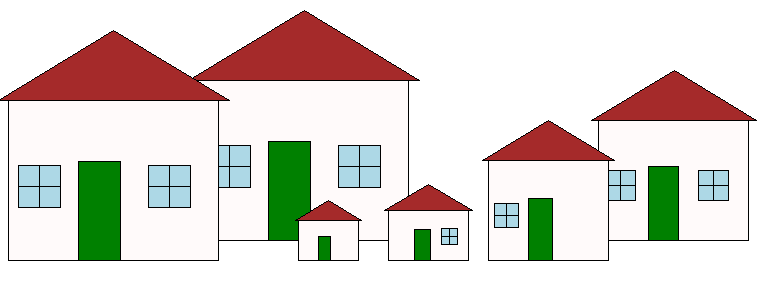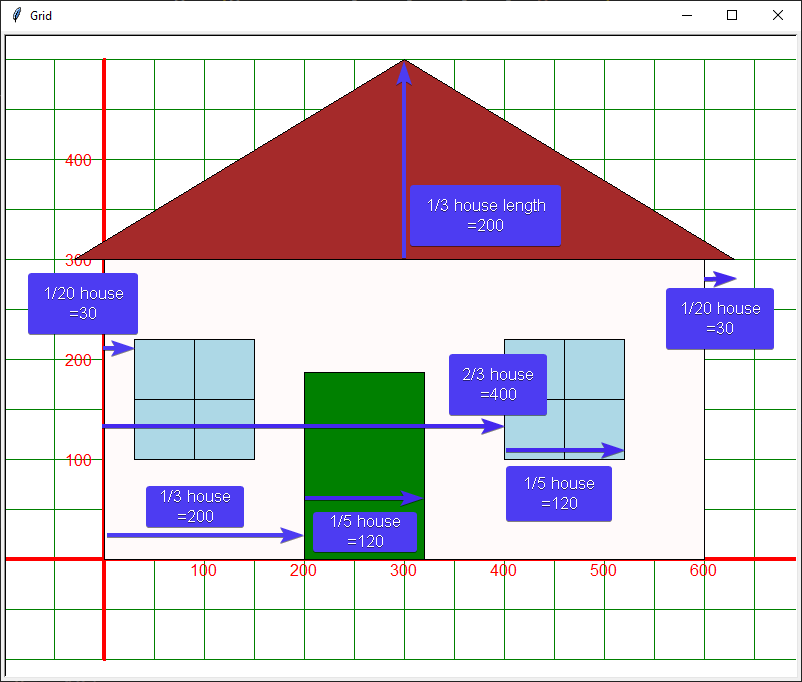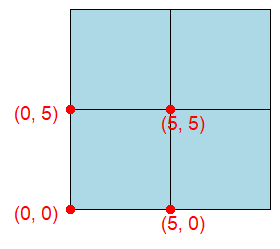# 14. Turtle houses module

Make a house module that provides definitions to make prefabricated houses with one line of code.
The houses are built from squares, rectangles and triangles.
To reduce the code in the main file, separate modules are used which group the code together.
Definitions for the base shapes, squares, rectangles and triangles, are placed in a module, `shapes.py`.
Definitions for house shapes will be placed in a module, `houses.py`.
Download the python file `shapes.py module`
Create the module, `houses.py`, by following the steps below to build a basic house shape by combining together various shapes from the shapes module.## 14.1. Importing the shapes module

The code below shows importing the shapes module as an alias via `import shapes as sh`.
This makes it shorter to refer to functions in the shapes module.
Instead of needing `shapes.rectangle`, only `sh.rectangle` is needed.
```import turtle
import shapes as sh
```

## 14.2. Houses

The diagram below shows the layout of a house with windows.
Positions and sizes of each shape are related to the position and sizes of the house.
Measurements are calculated using multiplication and division so that the house can be scaled up or down and still keep the same proportions.## 14.3. House definition

Write a definition that produces a house of given length, height and position with 0 to 2 windows.
house(t, length=60, height=40, start_pos=(0, 0), w_sides=None))
draw a house with 0-2 windows
t (class turtle.Turtle): turtle instance.
length (int, optional): length of house. Defaults to 60.
height (int, optional): height of house. Defaults to 40.
start_pos (tuple, optional): bottom left of house. Defaults to (0, 0).
w_sides (str, optional): L for windows on left side of house; R for right; LR for both. Defaults to None.
Use the functions from the `shapes.py` module to build the house function.
The code below is starter code for the house definition.
Comments are in place to indicate where each part of the house will be added.
```import turtle
import shapes as sh

def house(t, length=60, height=40, start_pos=(0, 0), w_sides=None):
"""draw a house with 0-2 windows

Args:
t (class turtle.Turtle): turtle instance.
length (int, optional): length of house. Defaults to 60.
height (int, optional): height of house. Defaults to 40.
start_pos (tuple, optional): bottom left of house. Defaults to (0, 0).
w_sides (str, optional): window sides - L for left side of house; R for right and LR for both. Defaults to None.
"""
# front of house

# door

# roof

# windows
```

## 14.4. Front of house

The front of the house will be a rectangle.
The length, width, start_pos of the rectangle are those of the house.
For simplicity, the pensize has been set to 1, pencolor to black and fillcolor to snow.
Use: `sh.rectangle(t, length=length, width=height, start_pos=start_pos, penw=1, penc="black", fillc="snow")`

Code Completion: front of house

Add the front of the house to the `house` definition.
```import turtle
import shapes as sh

def house(t, length=60, height=40, start_pos=(0, 0), w_sides=None):
"""draw a house with 0-2 windows

Args:
t (class turtle.Turtle): turtle instance.
length (int, optional): length of house. Defaults to 60.
height (int, optional): height of house. Defaults to 40.
start_pos (tuple, optional): bottom left of house. Defaults to (0, 0).
w_sides (str, optional): L for left side of house; R for right and LR for both. Defaults to None.
"""
# front of house
'''add code here to draw the rectangle'''

# door

# roof

# windows
```
Added the front of the house to the `house` definition.
```import turtle
import shapes as sh

def house(t, length=60, height=40, start_pos=(0, 0), w_sides=None):
"""draw a house with 0-2 windows

Args:
t (class turtle.Turtle): turtle instance.
length (int, optional): length of house. Defaults to 60.
height (int, optional): height of house. Defaults to 40.
start_pos (tuple, optional): bottom left of house. Defaults to (0, 0).
w_sides (str, optional): L for left side of house; R for right and LR for both. Defaults to None.
"""
# front of house
sh.rectangle(t, length=length, width=height, start_pos=start_pos,
penw=1, penc="black", fillc="snow")

# door

# roof

# windows
```
Test the code so far using:
`house(t, length=600, height=300, start_pos=(-300, -200), w_sides="LR")`
This will build a house of 600 by 300 at (-300, -200).
Only the houses main rectangle will be drawn so far.

1. Test the code so far.

```import turtle
import shapes as sh

s = turtle.Screen()
s.bgcolor("white")
s.title("Houses")
s.setup(width=800, height=600, startx=200, starty=100)

t = turtle.Turtle()
t.speed(9)

def house(t, length=60, height=40, start_pos=(0, 0), w_sides=None):
"""draw a house with 0-2 windows

Args:
t (class turtle.Turtle): turtle instance.
length (int, optional): length of house. Defaults to 60.
height (int, optional): height of house. Defaults to 40.
start_pos (tuple, optional): bottom left of house. Defaults to (0, 0).
w_sides (str, optional): L for left side of house; R for right and LR for both. Defaults to None.
"""
# front of house
sh.rectangle(t, length=length, width=height, start_pos=start_pos,
penw=1, penc="black", fillc="snow")

# door

# roof

# windows

house(t, length=600, height=300, start_pos=(-300, -200), w_sides="LR")

s.exitonclick()
```

## 14.5. Door of house

The door of the house will be a rectangle.

Code Completion: door of house

Complete the `house_door` definition.
```def house_door(t, length, height, start_pos):
"""draw door 1/3 along length of house, 1/5 of length of house, 1/1.6 of height of house

Args:
t (class turtle.Turtle): turtle instance.
length (int, optional): length of house.
height (int, optional): height of house.
start_pos (tuple, optional): bottom left of house.
"""
d_pos = (start_pos + length//XXX, start_pos)
d_height = height//XXX
d_length = length//XXX
sh.XXX(t, length=d_length, width=d_height, start_pos=d_pos, penw=1, penc="black", fillc="green")
```
Completed `house_door` definition.
```def house_door(t, length, height, start_pos):
"""draw door 1/3 along length of house, 1/5 of length of house, 1/1.6 of height of house

Args:
t (class turtle.Turtle): turtle instance.
length (int, optional): length of house.
height (int, optional): height of house.
start_pos (tuple, optional): bottom left of house.
"""
d_pos = (start_pos + length//3, start_pos)
d_height = height//1.6
d_length = length//5
sh.rectangle(t, length=d_length, width=d_height, start_pos=d_pos, penw=1, penc="black", fillc="green")
```
Test the code so far using:
`house(t, length=600, height=300, start_pos=(-300, -200), w_sides="LR")`
This will build a house of 600 by 300 at (-300, -200).
Only the houses main rectangle and door will be drawn.

1. Test the code so far

```import turtle
import shapes as sh

s = turtle.Screen()
s.bgcolor("white")
s.title("Houses")
s.setup(width=800, height=600, startx=200, starty=100)

t = turtle.Turtle()
t.speed(9)

def house_door(t, length, height, start_pos):
"""draw door 1/3 along length of house, 1/5 of length of house, 1/1.6 of height of house

Args:
t (class turtle.Turtle): turtle instance.
length (int, optional): length of house.
height (int, optional): height of house.
start_pos (tuple, optional): bottom left of house.
"""
d_pos = (start_pos + length//3, start_pos)
d_height = height//1.6
d_length = length//5
sh.rectangle(t, length=d_length, width=d_height, start_pos=d_pos, penw=1, penc="black", fillc="green")

def house(t, length=60, height=40, start_pos=(0, 0), w_sides=None):
"""draw a house with 0-2 windows

Args:
t (class turtle.Turtle): turtle instance.
length (int, optional): length of house. Defaults to 60.
height (int, optional): height of house. Defaults to 40.
start_pos (tuple, optional): bottom left of house. Defaults to (0, 0).
w_sides (str, optional): L for left side of house; R for right and LR for both. Defaults to None.
"""
# front of house
sh.rectangle(t, length=length, width=height, start_pos=start_pos,
penw=1, penc="black", fillc="snow")
# door
house_door(t, length, height, start_pos)

# roof

# windows

house(t, length=600, height=300, start_pos=(-300, -200), w_sides="LR")

s.exitonclick()
```

## 14.6. Roof of house

The roof of the house will be an isosceles triangle.

Code Completion: roof of house

Complete the `house_roof` definition.
```def house_roof(t, length, height, start_pos):
"""draw roof height 1/3 of house length
with overhang of 1/20 length of house over left side and right side of house

Args:
t (class turtle.Turtle): turtle instance.
length (int, optional): length of house.
height (int, optional): height of house.
start_pos (tuple, optional): bottom left of house.
"""
r_height = length//XXX
r_length = length * XXX
r_overhang = length//XXX
r_pos = (start_pos - r_overhang, start_pos + height)
sh.XXX(t, base=r_length, height=r_height, start_pos=r_pos, penw=1, penc="black", fillc="brown")
```
Complete the `house_roof` definition.
```def house_roof(t, length, height, start_pos):
"""draw roof with overhang of 1/20 length of house over left side and right side of house

Args:
t (class turtle.Turtle): turtle instance.
length (int, optional): length of house.
height (int, optional): height of house.
start_pos (tuple, optional): bottom left of house.
"""
r_height = length//3
r_length = length * 1.1
r_overhang = length//20
r_pos = (start_pos - r_overhang, start_pos + height)
sh.isosceles(t, base=r_length, height=r_height, start_pos=r_pos, penw=1, penc="black", fillc="brown")
```
Test the code so far using:
`house(t, length=600, height=300, start_pos=(-300, -200), w_sides="LR")`
This will build a house of 600 by 300 at (-300, -200).
Only the houses main rectangle and door and roof will be drawn.

1. Test the code so far

```import turtle
import shapes as sh

s = turtle.Screen()
s.bgcolor("white")
s.title("Houses")
s.setup(width=800, height=600, startx=200, starty=100)

t = turtle.Turtle()
t.speed(9)

def house_door(t, length, height, start_pos):
"""draw door 1/3 along length of house, 1/5 of length of house, 1/1.6 of height of house

Args:
t (class turtle.Turtle): turtle instance.
length (int, optional): length of house.
height (int, optional): height of house.
start_pos (tuple, optional): bottom left of house.
"""
d_pos = (start_pos + length//3, start_pos)
d_height = height//1.6
d_length = length//5
sh.rectangle(t, length=d_length, width=d_height, start_pos=d_pos, penw=1, penc="black", fillc="green")

def house_roof(t, length, height, start_pos):
"""draw roof with overhang of 1/20 length of house over left side and right side of house

Args:
t (class turtle.Turtle): turtle instance.
length (int, optional): length of house.
height (int, optional): height of house.
start_pos (tuple, optional): bottom left of house.
"""
r_height = length//3
r_length = length * 1.1
r_overhang = length//20
r_pos = (start_pos - r_overhang, start_pos + height)
sh.isosceles(t, base=r_length, height=r_height, start_pos=r_pos, penw=1, penc="black", fillc="brown")

def house(t, length=60, height=40, start_pos=(0, 0), w_sides=None):
"""draw a house with 0-2 windows

Args:
t (class turtle.Turtle): turtle instance.
length (int, optional): length of house. Defaults to 60.
height (int, optional): height of house. Defaults to 40.
start_pos (tuple, optional): bottom left of house. Defaults to (0, 0).
w_sides (str, optional): L for left side of house; R for right and LR for both. Defaults to None.
"""
# front of house
sh.rectangle(t, length=length, width=height, start_pos=start_pos,
penw=1, penc="black", fillc="snow")
# door
house_door(t, length, height, start_pos)

# roof
house_roof(t, length, height, start_pos)

# windows

house(t, length=600, height=300, start_pos=(-300, -200), w_sides="LR")

s.exitonclick()
```

## 14.7. windows

Combine 4 squares to make a 4 pane square window.Code Completion: roof of house

Complete the `house_window4` definition.
```def house_window4(t, length, height, start_pos, w_side, fillc="light blue"):
"""draw a 4 pane square window.
each window pane is half the total length of the window.
right hand window; 1/5 of house length; 2/3 of house length from left of house up 1/3 of height of house
left hand window; 1/5 of house length; 1/20 of house length from left of house up 1/3 of height of house

Args:
t (class turtle.Turtle): turtle instance.
length (int, optional): length of house.
height (int, optional): height of house.
start_pos (tuple, optional): bottom left of house.
w_side (str): L for left side of house; R for right and LR for both.
fillc (str, optional): window pane colour. Defaults to "light blue".
"""
w_length = length//XXX
if "L" in w_side:
w_pos = (start_pos + length//XXX, start_pos + height//XXX)
elif "R" in w_side:
w_pos = (start_pos + 2*length//XXX, start_pos + height//XXX)
else:
w_pos = (start_pos + length//XXX, start_pos + height//XXX)

sh.square(t, length=w_length, start_pos=w_pos, fillc="light blue")
sh.square(t, length=w_length, start_pos=(w_pos + w_length, w_pos), fillc="light blue")
sh.square(t, length=w_length, start_pos=(w_pos, w_pos + w_length), fillc="light blue")
sh.square(t, length=w_length, start_pos=(w_pos + w_length, w_pos + w_length), fillc="light blue")
```
Completed `house_window4` definition.
```def house_window4(t, length, height, start_pos, w_side, fillc="light blue"):
"""draw a 4 pane square window.
each window pane is half the total length of the window.
right hand window; 1/5 of house length; 2/3 of house length from left of house up 1/3 of height of house
left hand window; 1/5 of house length; 1/20 of house length from left of house up 1/3 of height of house

Args:
t (class turtle.Turtle): turtle instance.
length (int, optional): length of house.
height (int, optional): height of house.
start_pos (tuple, optional): bottom left of house.
w_side (str): L for left side of house; R for right and LR for both.
fillc (str, optional): window pane colour. Defaults to "light blue".
"""
w_length = length//10
if "L" in w_side:
w_pos = (start_pos + length//20, start_pos + height//3)
elif "R" in w_side:
w_pos = (start_pos + 2*length//3, start_pos + height//3)
else:
w_pos = (start_pos + length//20, start_pos + height//3)

sh.square(t, length=w_length, start_pos=w_pos, fillc="light blue")
sh.square(t, length=w_length, start_pos=(w_pos + w_length, w_pos), fillc="light blue")
sh.square(t, length=w_length, start_pos=(w_pos, w_pos + w_length), fillc="light blue")
sh.square(t, length=w_length, start_pos=(w_pos + w_length, w_pos + w_length), fillc="light blue")
```

## 14.8. Houses with windows

Test the completed code using:
`house(t, length=600, height=300, start_pos=(-300, -200), w_sides="LR")`
This will build a house of 600 by 300 at (-300, -200).
Only the houses main rectangle and door and roof will be drawn.

1. Test the completed code

```import turtle
import shapes as sh

s = turtle.Screen()
s.bgcolor("white")
s.title("Houses")
s.setup(width=800, height=600, startx=200, starty=100)

t = turtle.Turtle()
t.speed(9)

def house_door(t, length, height, start_pos):
"""draw door 1/3 along length of house, 1/5 of length of house, 1/1.6 of height of house

Args:
t (class turtle.Turtle): turtle instance.
length (int, optional): length of house.
height (int, optional): height of house.
start_pos (tuple, optional): bottom left of house.
"""
d_pos = (start_pos + length//3, start_pos)
d_height = height//1.6
d_length = length//5
sh.rectangle(t, length=d_length, width=d_height, start_pos=d_pos, penw=1, penc="black", fillc="green")

def house_roof(t, length, height, start_pos):
"""draw roof with overhang of 1/20 length of house over left side and right side of house

Args:
t (class turtle.Turtle): turtle instance.
length (int, optional): length of house.
height (int, optional): height of house.
start_pos (tuple, optional): bottom left of house.
"""
r_height = length//3
r_length = length * 1.1
r_overhang = length//20
r_pos = (start_pos - r_overhang, start_pos + height)
sh.isosceles(t, base=r_length, height=r_height, start_pos=r_pos, penw=1, penc="black", fillc="brown")

def house_window4(t, length, height, start_pos, w_side, fillc="light blue"):
"""draw a 4 pane square window.
each window pane is half the total length of the window.
right hand window; 1/5 of house length; 2/3 of house length from left of house up 1/3 of height of house
left hand window; 1/5 of house length; 1/20 of house length from left of house up 1/3 of height of house

Args:
t (class turtle.Turtle): turtle instance.
length (int, optional): length of house.
height (int, optional): height of house.
start_pos (tuple, optional): bottom left of house.
w_sides (str): L for left side of house; R for right and LR for both.
fillc (str, optional): window pane colour. Defaults to "light blue".
"""
w_length = length//10
if "L" in w_side:
w_pos = (start_pos + length//20, start_pos + height//3)
elif "R" in w_side:
w_pos = (start_pos + 2*length//3, start_pos + height//3)
else:
w_pos = (start_pos + length//20, start_pos + height//3)

sh.square(t, length=w_length, start_pos=w_pos, fillc="light blue")
sh.square(t, length=w_length, start_pos=(w_pos + w_length, w_pos), fillc="light blue")
sh.square(t, length=w_length, start_pos=(w_pos, w_pos + w_length), fillc="light blue")
sh.square(t, length=w_length, start_pos=(w_pos + w_length, w_pos + w_length), fillc="light blue")

def house(t, length=60, height=40, start_pos=(0, 0), w_sides=None):
"""draw a house with 0-2 windows

Args:
t (class turtle.Turtle): turtle instance.
length (int, optional): length of house. Defaults to 60.
height (int, optional): height of house. Defaults to 40.
start_pos (tuple, optional): bottom left of house. Defaults to (0, 0).
w_sides (str, optional): L for left side of house; R for right and LR for both. Defaults to None.
"""
# front of house
sh.rectangle(t, length=length, width=height, start_pos=start_pos,
penw=1, penc="black", fillc="snow")
# door
house_door(t, length, height, start_pos)

# roof
house_roof(t, length, height, start_pos)

# windows
if w_sides is not None:
if "R" in w_sides:
# right hand window
house_window4(t, length, height, start_pos, "R")
if "L" in w_sides:
# left hand window
house_window4(t, length, height, start_pos, "L")

house(t, length=600, height=300, start_pos=(-300, -200), w_sides="LR")

s.exitonclick()
```

## 14.9. Multiple housesUse the completed house definition to make a row of houses of different sizes.

Exercises

1. Test the house module using the code below.

2. Make your own row of houses of different sizes.

```import turtle
import houses as h

s = turtle.Screen()
s.bgcolor("white")
s.title("Houses")
s.setup(width=800, height=600, startx=200, starty=100)
s.tracer(0, 0)

t = turtle.Turtle()
t.speed(0)

h.house(t, length=210, height=160, start_pos=(-200, 20), w_sides="LR")
h.house(t, length=150, height=120, start_pos=(200, 20), w_sides="LR")
h.house(t, length=60, height=40, start_pos=(-100, 0), w_sides=None)
h.house(t, length=80, height=50, start_pos=(-10, 0), w_sides="R")
h.house(t, length=120, height=100, start_pos=(90, 0), w_sides="L")
h.house(t, length=210, height=160, start_pos=(-390, 0), w_sides="LR")

t.ht()
s.update()
s.exitonclick()
```